# The mess converting decibels to voltages in test instruments (dBm, dBW, W, dbV, V)

Complex conversions between decibels and physical quantity has always been a rich source of confusion. The reason is that dB(something) is actually a loaded word with hidden assumptions:

• dB always works on base-10
• dB is always a relative (dimensionless) POWER quantity, the convenience scaling factor is always 10. It does NOT make sense directly on non-power quantities.
• dB(something) is always with respect to a quantity (the something), and the reference quantity is often not written in full. Since there is an implicit reference, db(something) can be mapped to absolute quantities.

If you are a diverse multi-disciplinary techie like me (math, electronics, programming, computers), it’d frustrate the hell out of you when you talk to people who has been working exclusively on a narrow field for at least a decade and they have a table of commonly used numbers in their memorized: they act like you are supposed to know how to get the numbers in the dB-variant that they use, than explaining to you what the field-specific assumptions are (likely because they forgot about it).

I hope this post will clear up the confusion by working out an example in test instrumentation, most commonly in RF as well, converting dBm to Volts.

Before I start, I’ll clarify the most common form of beginner confusion in EE and physics: converting between dB and voltages: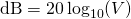This looks like a definition of decibel, except the scaling factor is 20 magically for Volts. It is correct (under very commonly used assumptions) as well. Most people take it as an equivalent definition of decibels, and throw away these important assumptions behind it:

• the reference is 1V,
• and the resistance* (common to the voltage of interest and the reference voltage) gets cancelled

and run into troubles when they venture into those dB-variants like dBm. Technically the above should be written as dBV, but I have seen very few people use the clearer term.

The decibel formula for voltage came from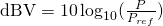where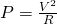and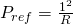, you get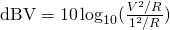The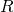get cancelled out and you get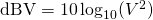People moved the squaring out and lumped (multiplied) it with the scaling factor 10: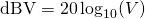So the whole reason why it is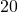instead of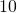is simply because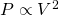, and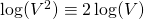.

Now back to the business converting dBm to dBV or Volts.

First of all dBm is dB(mW), NOT dB(mV). The RF/telecom people are just too lazy to write out the most important part: the physical quantity expressly, because nearly all the time, it’s the power that matters to them.

However, I often need to connect a RF generator to a high bandwidth oscilloscope, so the very self-centered RF/telecom nomenclature start to become problematic when people of different fields need to talk to each other. Oscilloscope see everything in volts. RF sees everything in power, often in dB.

Then we get to the (mW) part, which means the reference quantity in the definition is 1mW, which is a physical quantity with dimensions. Then how are we going to convert it to Volts? You cannot jump to the shortcut formula I illustrated above with the 20 factor this time because the reference is in mW and your quantity is in Volts.

You’ll need to convert power to voltages. To do so, you’ll need to know voltages induced by power ‘dissipated’ through a ‘resistance’ across a component (load). The missing gap is that you will need to know the load ‘resistance’ before the conversion. With that, you can use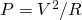, or rewritten as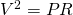when it’s more convenient.

All RF-related test-instruments and bench function generators typically have a 50Ω output impedance, which means it also assumes a matching 50Ω as mathematically, it provides the maximum power transfer (sadly split evenly between the load and wasted at the instrument’s output impedance). For convenience, the amplitude you see in the instrument control panel refers to the amplitude you see at a 50Ω load, not what the instrument pumps out internally (that’s why you see 2Vpp when your function generator says 1Vpp if you hook it up to a low-end oscilloscope that serves 1MΩ by default).

Since we are dealing with continuous wave (not transient power), all amplitude quantities on RF test instruments are in RMS (power or voltage) unless otherwise specified. So the quantities we have for dBm is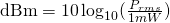when written in terms of voltages,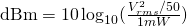Instead of splitting it into 3 terms and immediately grouping the constants, I’d like to first convert dBm to dBW: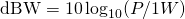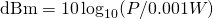The linear quantity in dBm is artificially scaled 1000 times bigger than in dbW, to put it in a comfortable scale for us to work with smaller signals. Therefore dBm is always 30dB higher than dbW (the smaller the reference, the bigger the relative numbers look).

So back to the above in dBW, we subtract 30dB to get to dBW: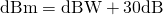where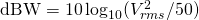We can separate the load and put it on the left hand side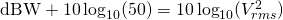The right hand side is dBV, and you can think of the load as scaling the power up (inducing) the voltage-squared quantity (, or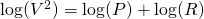).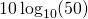is 16.9897dB, for most purposes I’ll just say the load lift the dBW by 17dB when turning it into dbV.

Having both together,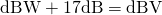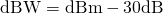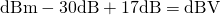(This is how you should remember it, so you can replace the +17dB for 50Ω with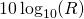when you work on other applications, like 600Ω, 4Ω, 8Ω for audio.)

Basically:

-30dB to undo the mili- prefix (small reference value bloated the numbers)
+17dB to account for the load inducing the voltage by burning Watts

The end result (for the 50Ω case):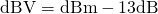Then you can convert dBV to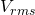: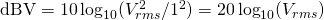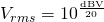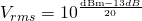Phew! That’s a lot of steps to get to something this simple. So the moral of the story is that these assumptions cannot be ignored:

• The quantity is always power in dB, not voltages
• dB(mW) has a reference of 1mW. The smaller the reference, the bigger the numbers
• RMS voltages and power are used in RF
• 50Ω is the load required to convert from power to voltages

Keysight already has a derivation, but it’s just a bunch of equations. The missing gap I want to fill in this blog post is that people find this so confusing they’d rather believe a formula or a table pulled on the internet:  it doesn’t have to be this way after realizing that there’s a bunch of overlooked assumptions.

* Technically I should call it (load) impedance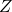, as in RF, capacitive and inductive elements are nearly always involved, but I want to make it appealing to those with high school physics background.

619 total views,  1 views today